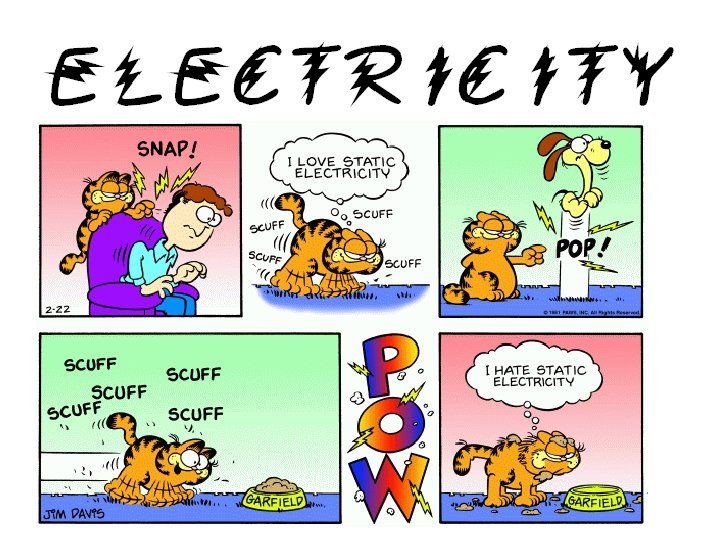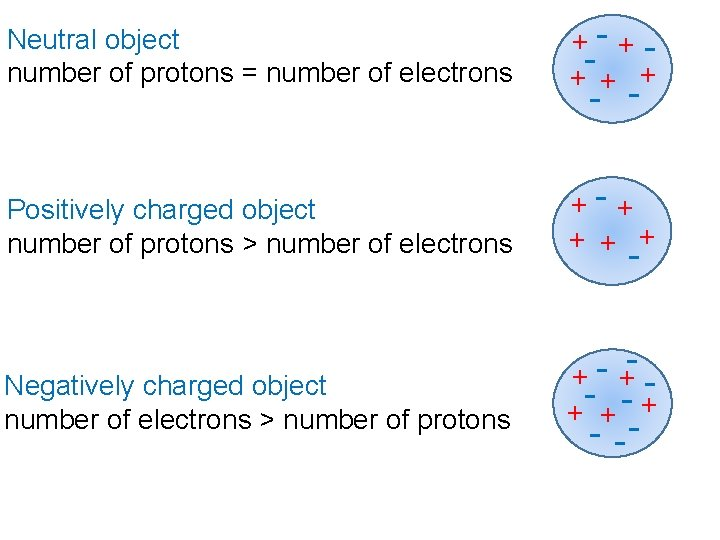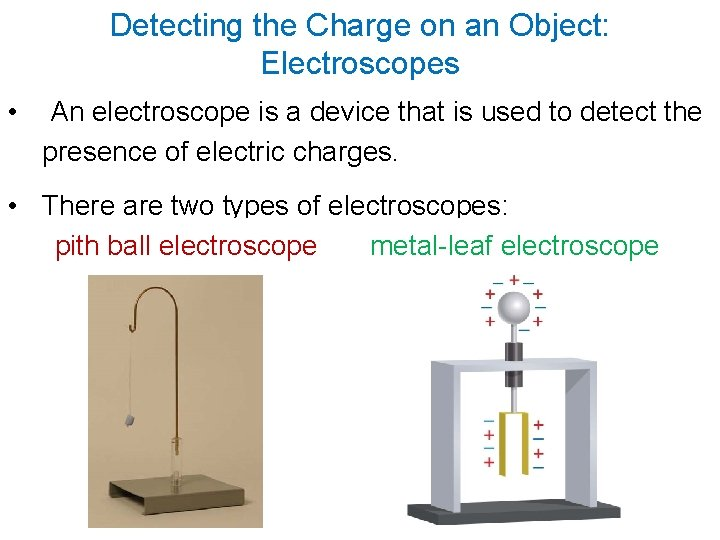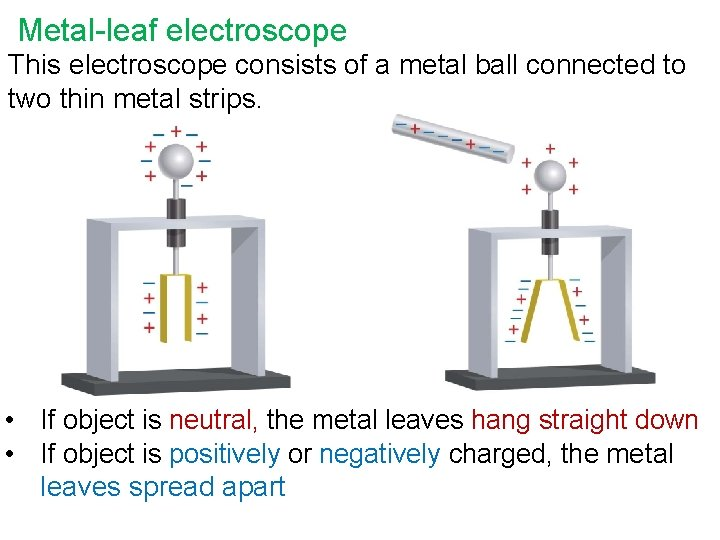# What is Static Electricity Static electricity is an

• Slides: 9What is Static Electricity? Static electricity is an imbalance of positive and negative charges on an object. Charges on Objects • An object may be positively charged, negatively charged, or neutral. • An object becomes charged when electrons are either removed from or added to the object.Neutral object number of protons = number of electrons +- ++ + + Positively charged object number of protons > number of electrons +- + + Negatively charged object number of electrons > number of protons + ++ +- + - -- -Law of Electric Charges • A charged object exerts an electric force on a nearby object. • The force either attracts or repels the nearby object. The Law of Electric Charges states that: • opposite charges attract each other • objects with like charges repel each other • charged objects will attract neutral objectsDetecting the Charge on an Object: Electroscopes • An electroscope is a device that is used to detect the presence of electric charges. • There are two types of electroscopes: pith ball electroscope metal-leaf electroscopeWith an electroscope you can determine if an object is neutral or if the object has a charge on it. You do this by observing what happens when the object is brought close to a neutral electroscope.Pith ball electroscope This electroscope consists of a small ball made of pith or cork or Styrofoam hanging from a thread. • If object is neutral, the pith ball does not move • If object is positively or negatively charged, the pith ball swings toward objectMetal-leaf electroscope This electroscope consists of a metal ball connected to two thin metal strips. • If object is neutral, the metal leaves hang straight down • If object is positively or negatively charged, the metal leaves spread apartHomework p 471 #3 -9